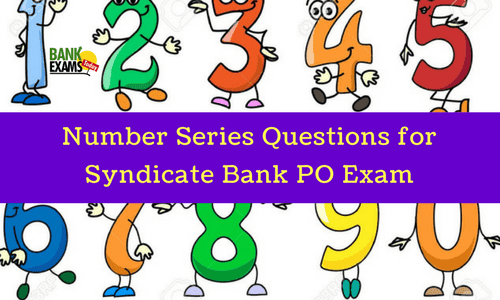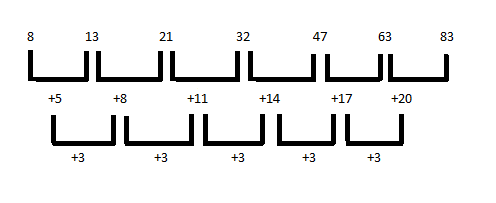# Number Series Quiz: Set 15Directions 1-4: What will come in the place of question mark (?) in the following number series?

1) 6, 16, 36, 76, 156, ?
(a) 160
(b) 253
(c) 316
(d) 402
(e) None of these

2) 325, 259, 204, 160, ?
(a) 105
(b) 125
(c) 135
(d) 127
(e) 138

3) 53, 53, 40, 40, 27, 27, ?
(a) 13
(b) 14
(c) 27
(d) 53
(e) 40

4) 45, 54, 47, ?, 49, 56, 51, 57, 53
(a) 48
(b) 46
(c) 52
(d) 55
(e) 63

Directions 5-9: In Each of the following questions, one term in the number series in Wrong. Find out the Wrong term.

5) 125, 126, 124, 127, 123, 129
(a) 126
(b) 124
(c) 127
(d) 123
(e) 129

6) 3, 4, 10, 32, 136, 685, 4116
(a) 32
(b) 10
(c) 3
(d) 685
(e) 4116

7) 3, 2, 8, 9, 13, 22, 18, 32, 23, 42
(a) 3
(b) 9
(c) 13
(d) 8
(e) 23

8) 2, 5, 10, 50, 500, 5000
(a) 5000
(b) 500
(c) 50
(d) 10
(e) 2

9) 8, 13, 21, 32, 47, 63, 83
(a) 13
(b) 21
(c) 32
(d) 47
(e) 63
(f) 83

### Answers :

1. Solution :
Hence,? = 316
The correct answer is (C).

2. Solution :
Hence,? = 127
The correct answer is (C).

3. Solution :
Hence,? = 14
The correct answer is (B)

4. Solution :
Here the given series is combination of two series:
Series I : 45, 47, 49, 51, 53
Series II : 54, ?, 56, 57
Now, from series II
The correct answer is (D).

5. Solution:
Hence, 129 is Wrong term.
The correct answer is (E).

6. Solution:
Hence, 32 is Wrong number.
The correct answer is (A).

7. Solution:
Here, given series is combination of two series.
Series I: 3, 8, 13, 18, 23
Series II: 2, 9, 22, 32, 42
In series I,
In series II,
Hence, 9 is Wrong number.
The correct answer is (B).

8. Solution: Here,
2×5 = 10
5×10 = 50
10×5 = 50
50×500 = 25000
Hence, 5000 is Wrong term.
The correct answer is (A).

9. Solution:Hence, 47 is Wrong number.
The correct answer is (D).

Free 100 Number Series Questions PDF link#### What's trending in BankExamsToday

Smart Prep Kit for Banking Exams by Ramandeep Singh - Download here

##### Join 40,000+ readers and get free notes in your email#### Post a Comment

Thanks for commenting. Follow us on Telegram. Search BankExamsToday